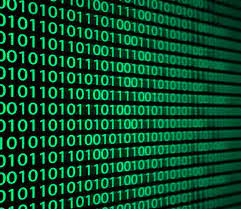# Learning Python with Advent of Code Walkthroughs

Dazbo's Advent of Code solutions, written in Python

# The Python Journey - Working with Binary and Other BasesIf you’re not familiar with binary, hexadecimal, and bases in general, then I’d suggest you first checkout this friendly page.

## Converting Decimal to Other Bases

``````a_num = 20

bin_as_str = bin(20)
print(f"bin_as_str: {bin_as_str}")
print(f"bin component: {bin_as_str[2:]}")

oct_as_str = oct(20)
print(f"bin_as_str: {oct_as_str}")
print(f"bin component: {oct_as_str[2:]}")

hex_as_str = hex(20)
print(f"bin_as_str: {hex_as_str}")
print(f"bin component: {hex_as_str[2:]}")
``````

Output:

``````bin_as_str: 0b10100
bin component: 10100
bin_as_str: 0o24
bin component: 24
bin_as_str: 0x14
bin component: 14
``````

## Converting Any Base to Decimal

``````# bin to dec
print(int("01101001", 2))
print(int("0b01101001", 2))

# hex to dec
print(int("ff", 16))
``````

Output:

``````105
105
255
``````

## Converting from Hex to Binary

``````hex_str = "7bff9"

# To get bin value without leading zeroes, just convert from hex to dec - with int(),
# and then from dec to bin - with bin()
bin_val = bin(int(hex_str, 16))
print(bin_val)

# And now using the 'b' format specifier to go from dec to bin with leading zeroes
bin_len = 4*len(hex_str)
bin_val = "{0:0{width}b}".format(int(hex_str, 16), width=bin_len)
print(bin_val)
``````

The first conversion is just using the int() and bin() functions that we’ve already seen.

The second conversion:

• First converts from hex to decimal, using `int(value, base)`, where `base=16`.
• Then converts the decimal number to binary, by applying a `format spec`, where:
• The first `{0}` is the first parameter to the `format()` method, i.e. the decimal value;
• And `:0{width}b` tells `format()` to convert to `binary` type, and then pad with `0` in order to achieve the desired overall length.

In general, when using a format spec, the format is:

``````{fill}{align}{width}{type}
``````

Output:

``````0b1111011111111111001
01111011111111111001
``````

## Bit Operations

Operation Operator Example
Bit shift left y places x « y Each shift left results in a doubling of the value. E.g. 0b110 < 2 = 0b11000
Bit shift right y places x » y E.g. shift right results in an integer division by 2. E.g. 0b11000 » 3 = 0b11
Bitwise AND x & y 1 only if both are 1.
Bitwise OR x | y 1 if either is 1
Complement / NOT ~x Switch each 0 for 1, and each 1 for 0.
Exclusive OR (XOR) x ^ y 1 where one or other, but not both

### Demo

``````BIN_VAL_AS_STR = "0b0110"
bin_val_as_dec = int(BIN_VAL_AS_STR, 2)
print(f"bin_val as str: {BIN_VAL_AS_STR}'; as decimal: {bin_val_as_dec}")

bin_digit_len = len(str(BIN_VAL_AS_STR)[2:])
print(f"\nbin digits length: {bin_digit_len}")

# This is useful for getting a binary number with leading zeros.
# Results in "#06b"
# The "#" means "include 0b prefix"
# The 0 means include leading zeros
# The 6 means total length of digits (including the 0b)
format_specifier = "#0"+str(int(bin_digit_len)+2)+"b"
print(f"Format specifier: {format_specifier}")

bin_val_as_bin_str = bin(int(BIN_VAL_AS_STR, 2))

print("\nOperations...")
shift_left = bin_val_as_dec << 2
print(f"shift {bin(bin_val_as_dec)} left by 2 as bin: {bin(shift_left)}; as dec: {shift_left}")

shift_right = shift_left >> 3
print(f"shift {bin(shift_left)} right by 3 as bin: {bin(shift_right)}; as dec: {shift_right}")

complement = ~bin_val_as_dec
print(f"complement of {BIN_VAL_AS_STR} as dec: {complement}")

complement_positive = ~bin_val_as_dec & int(ones_mask, 2)
print(f"Positive complement of {BIN_VAL_AS_STR} as bin: {bin(complement_positive)}")
print(f"Positive complement of {BIN_VAL_AS_STR} as dec: {complement_positive}")
``````

Output:

``````bin_val as str: 0b0110'; as decimal: 6

bin digits length: 4
Format specifier: #06b
The complement operation is worth noting. When we take the complement of 6, we end up with -7. This is because negative numbers - in Python - are represented by setting the most significant (leftmost) bit to 1. Thus, if we have a binary number that has a leading 0 and complement it, we will always end up with a negative number. But often that’s not what we want. A neat trick is to perform a bitwise `&` with the complement of a binary number, using a mask that is set to all 1s. If we do this, then we will successfully invert all the bits, but in the form of a positive number.